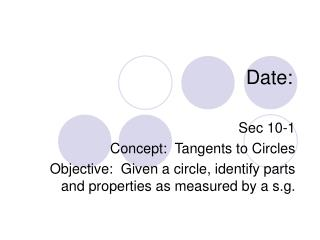DownloadDownload PresentationDate:

# Date:

Télécharger la présentation## Date:

- - - - - - - - - - - - - - - - - - - - - - - - - - - E N D - - - - - - - - - - - - - - - - - - - - - - - - - - -
##### Presentation Transcript

1. Date: Sec 10-1 Concept: Tangents to Circles Objective: Given a circle, identify parts and properties as measured by a s.g.

2. Circle Activity – Draw a diagram illustrating the words listed below. Identify each word on the illustration. LOOK IN CH 10 Circle Center Radius Diameter Chord Secant Tangent Tangent Circles Concentric Common Tangent Interior of a circle Exterior of a circle Point of Tangency Minor Arc Major Arc Inscribed Angle Central Angle

3. Example: The diagram shows the layout of the streets on Mexcaltitlan Island. 1. Name 2 secants 2. Name two chords 3. Is the diameter of the circle greater than HC? 4. If ΔLJK were drawn, one of its sides would be tangent to the circle. Which side is it?

4. Draw internal/external tangents Tangent Circles 2 points of intersection 1 point of intersection No points of intersection

5. P l QP  l Q Thm 10-1: If a line is tangent to a circle, then it is perpendicular to the radius drawn to the point of tangency. If l is tangent to circle Q at P, then

6. r A 16 r B C 24 Example: If BC is tangent to circle A, find the radius of the circle. Use the pyth. Thm. r2+242 = (r+16)2 r2+576 = (r+16)(r+16) r2+576 = r2+16r+16r+256 r2+576 = r2+32r+256 • 576 = 32r + 256 • -256 -256 • 320 = 32r • 32 • 10 = r

7. Example: A green on a golf course is in the shape of a circle. A golf ball is 8 feet from the edge of the green and 28 feet from a point of tangency on the green, as shown at the right. Assume that the green is flat. 1. What is the radius of the green 2. How far is the golf ball from the cup at the center?

8. P l If l  QP at P, then l is tangent to circle Q Q Thm 10-2: in a plane, if a line is perpendicular to a radius of a circle at its endpoint on the circle, then the line is tangent to the circle

9. E C 11 43 D 45 Example: Is CE tangent to circle D? Use the Pyth. Thm: 112+432 = 452 121+1849 = 2025 1970 ≠ 2025 No, it’s not tangent

10. R S P If SR and TS are tangent to circle P, then SR  TS T Thm: If 2 segments from the same exterior point are tangent to a circle, then they are congruent.

11. X2 - 4 B A C 21 D Example: AB and DA are tangent to circle C. Find x. X2 – 4 = 21 + 4 +4 X2 = 25 X=5

12. Statements Reasons 1. 2. 3. 4. 5. Seg. Addition Post. 6. • 1. • 2. PQTQ; QSQR • PQ=TQ; • QS=QR • 4. PQ+QS = TQ+QR • 5. • 6.

13. Today’s Work In Class: HW:

14. Tangent Center Radius Diameter Chord Secant Circle: A circle is the set of all points in a plane that are equidistant from a given point called the center of the circle. A circle with center P is called “circle P” or P